High School Chemistry : Using Stoichiometry in Solutions

Example Questions

Example Question #21 : Stoichiometry

What is the mass of the solid left over after boiling off 100mL of 0.4M NaCl solution?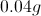None of the available answers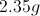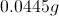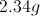Explanation:

The remaining mass with be equal to the mass of the sodium chloride in the solution. Once the solvent (water) evaporates, the solute will remain.

Atomic mass of sodium is ~23. Atomic mass of chlorine is ~35.5. Molecular mass of NaCl is ~58.5.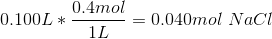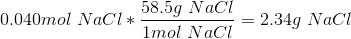Example Question #22 : Stoichiometry

How much water is necessary to make a solution with a molality of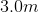when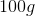of sodium chloride are in the solution?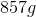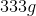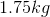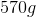Explanation:

This question is asking us to solve for molality, which has the following equation: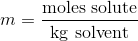Note how the equation does not ask for liters of solution like molarity, but instead requires the kilograms of solvent used in the solution. By dividing the mass of the sodium chloride by its molar mass, we can solve for the moles of sodium chloride in solution.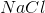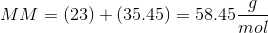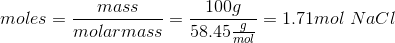This will allow us to solve for the mass of water used in the solution.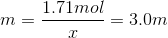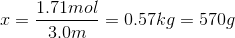This gives us the mass of water required to make the solution.

Example Question #2 : Using Stoichiometry In Solutions

Consider the reaction of potassium carbonate with calcium nitrate to form potassium nitrate and calcium carbonate: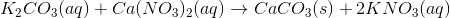Suppose 50ml of a 0.250M potassium carbonate solution was mixed with 100ml of a 0.175M calcium nitrate solution. What is the maximum amount of calcium carbonate that could be obtained?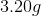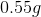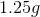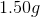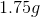Explanation:

First, we must determine how many moles of each reactant begin the reaction by multiplying the molarity by the volume. Don't forget to convert volume to liters!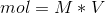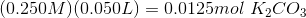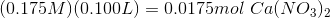Next, use the reaction coefficients (i.e. the stoichiometry) to determine how many moles of calcium carbonate could be formed from each of the reactants. In this case, there is a 1:1 molar ratio between both reactants and calcium carbonate.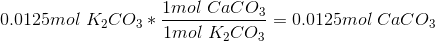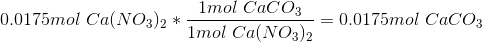Thus, 0.0125 moles of potassium carbonate could form 0.0125 moles of calcium carbonate, while 0.0175 moles of calcium nitrate could form 0.0175 moles of calcium carbonate. The maximum amount of product is going to be determined by the limiting reactant, i.e. the reactant that provides the least amount of product. In this case, the limiting reactant is potassium carbonate, and the maximum yield of calcium carbonate is 0.0125mol.

For the final step convert this value to grams: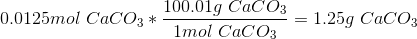All High School Chemistry Resources